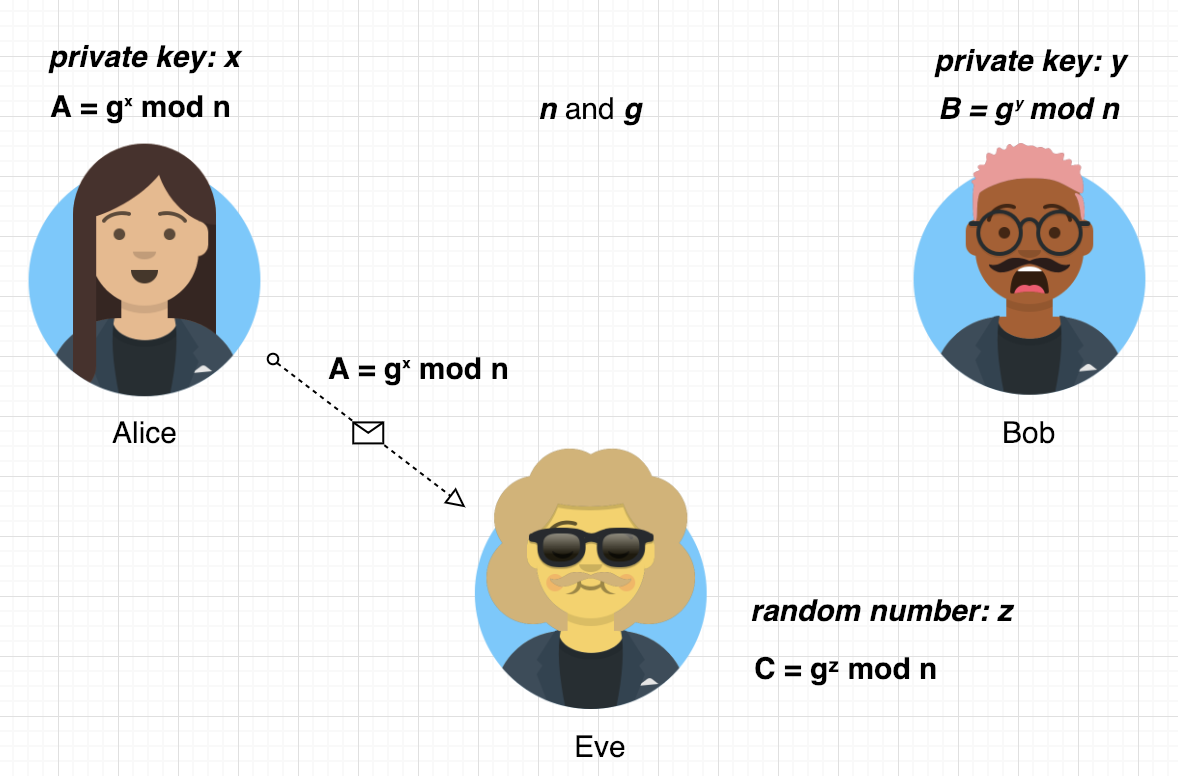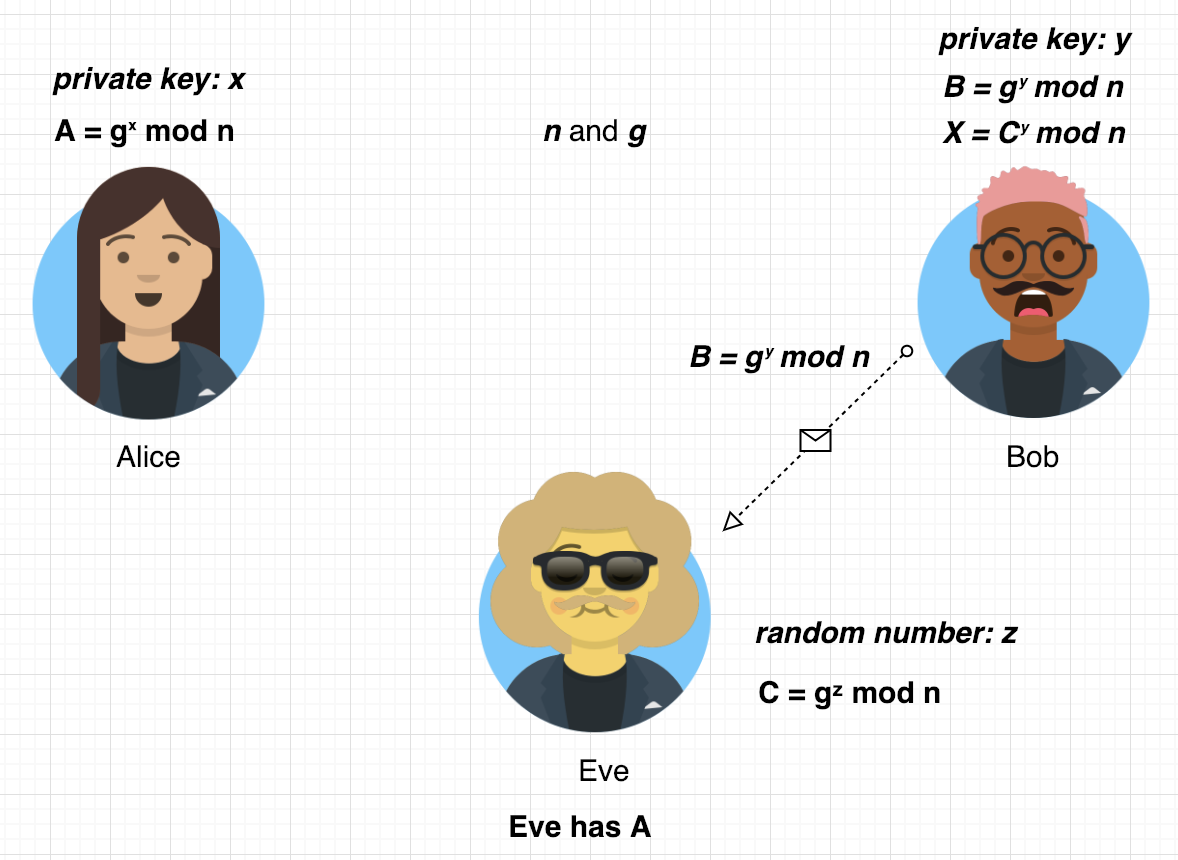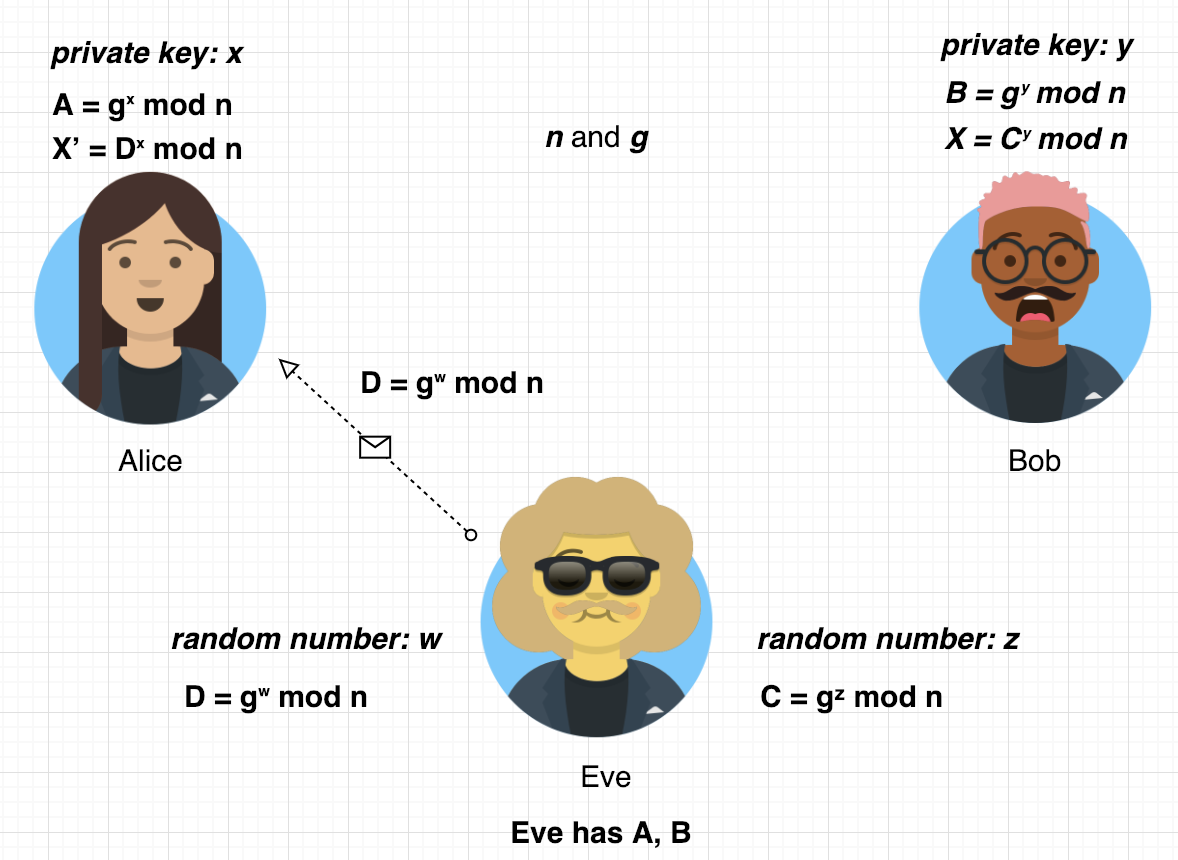Diffie-Hellman Key Exchange

One disadvantage of symmetric cryptosystems which require private key like AES is that the private key must be exchanged. And Diffie-Hellman key exchange algorithm enables exchange private keys over a public channel. So it can solves following dilemma.

Alice and Bob want to share a secret key which is going to be used in a symmetric cipher, but all of their communication channel are insecure, furthermore every infomation that is exchanged over channel is observed by their adversary. In this situation how could they share a key without making it available to their adversary?

And one of the solutions is Diffie-Hellman key exchange, and this is not about encryption or decryption but to securely exchange the private keys for symmetric cryptosystems.

How Algorithm Proceed?

1. First sender Alice generate huge prime numbers n and g, and it is best to choose numbers which g is the primitive root of n, typically both numbers are over 1024 bits. Then sender (Alice) sends it to the receiver (Bob). And everyone can see n and g.

Before explaining about why it is best to choose primitive root, what is primitive root?

primitive root

If g is the primitive root of n, then g mod n, g² mod ngⁿ⁻¹ mod n generates all the integers within the range [1, n-1]. For example, let’s take n=11 and g=8

mod 11 = 8
mod 11 = 9
mod 11 = 6
8⁴ mod 11 = 4
8⁵ mod 11 = 10
8⁶ mod 11 = 3
8⁷ mod 11 = 2
8⁸ mod 11 = 5
8⁹ mod 11 = 7
8¹⁰ mod 11 = 1

We can see that 8¹ mod 11, 8² mod 11, … 8¹⁰ mod 11 generates integers set which values are in the range [1, 10], so we can conclude that 8 is a primitive root of 11.

Then how about n=11 and g=10?

10¹ mod 11 = 10
10² mod 11 = 1
10³ mod 11 = 10
10⁴ mod 11 = 1
10⁵ mod 11 = 10
10⁶ mod 11 = 1
10⁷ mod 11 = 10
10⁸ mod 11 = 1
10⁹ mod 11 = 10
10¹⁰ mod 11 =1

In the case of n=11 and g=10, the result integer set is {1, 10} which is not all the integers within the range of [1, 10]. So 10 is not a primitive root of 11.

2. Both sender (Alice) and receiver (Bob) generate a random number which is less than n-1, let’s assume that Alice generates x and Bob generates y, and these values are going to be their private keys.

3. Alice calculates A = gˣ mod n with its own private key x, in the same way Bob calculates B = gʸ mod n with its own private key y, and send these to each other.

4. Alice and Bob then can calculate the shared secret key X:

• Alice calculates: Bˣ mod n = (gʸ mod n)ˣ mod n = gˣʸ mod n = X
• Bob calculates: Aʸ mod n = (gˣ mod n)ʸ mod n = gˣʸ mod n = X

Insight

And here’s important insight. Both Alice and Bob knows nothing about counterpart’s private key, but they can calculate same value.

One more important thing to know is that A can be calculated by Alice exclusively, B by Bob exclusively. And X includes both A and B.

As a result both of them know nothing about each other’s private key, but at the same time, they can calculate (share) a new secret key which include each private key.

Example

Let’s follow the algorithm with concrete number.

1. Alice generate huge prime number, in this example for simplicity, let’s assume n=37 and g=13, then sends these to Bob.
2. Both Alice and Bob generates its own private key. Now Alice generates x=23, Bob generates y=14.
3. Alice calculates A = 13²³ mod 37 = 2, Bob calculates B = 13¹⁴ mod 37 = 25. And send these values to each other.
4. Alice calculates Bˣ mod n, which is 25²³ mod 37 = 30, Bob calculates Aʸ mod n, which is 2¹⁴ mod 37 = 30, both calculates same value and now they can use this value for symmetric cryptosystem private key like AES.

Why choose primitive root for n and g?

The size of keyspace is crucial in cryptography, if there are few possible keys someone malicious can crack the system with brute-force attack quite fast. So we have to make the size of the keyspace as large as possible.

Let’s think about what would happen if we choose n and g and g is not primitive root of n. Take n=11 and g=10 as example (we talked 10 is not primitive root of 11).

Then when Alice and Bob calculates new private key X, they use gˣʸ mod n modular formula. But as you know g mod n result integer set is just {1, 10}, so there’s only two possible keys.

What about n=11 and g=8, g is primitive root of 11, we know g mod n result integer set is {1, 2 …. 10}, which is the maximum set.

Cracking the Diffie-Hellman Key Exchange

The Diffie-Hellman cryptosystem relies on the fact that there is no efficient algorithm to calculate the discrete logarithm.

The attacker may know n, g, A and B because those values are being sent over a public channel. But in the modular formula which calculates X, Aʸ mod n or Bˣ mod n, there’s no fast algorithm to calculate x or y.

So it is known that Diffie-Hellman cryptosystem is secure because of the fact that the discrete logarithm problem is extremely hard to solve.

Man in the middle attack

One problem in Diffie-Hellman key exchange algorithm is that there’s no authentication when exchanging n, g, A and B values. So attacker can use man-in-the-middle attack.

This is not about cracking the cryptosystem directly because it is practically impossible to solve discrete logarithm problem, but about exploiting the fact that Diffie-Hellman doesn’t provide any authentication.Alice send A to Eve, but Eve is the man in the middle
1. After Eve got A, Eve generate a random number z, which is smaller than n-1. And he is going to pretend to Bob that he is Alice.

2. Instead of sending A to Bob, Eve calculates gᶻ mod n = C, and sends C to Bob.Bob think X as shared secret key with Alice, but it’s not

3. At this point Bob thinks that he Cʸ mod n = X is the shared secret key with Alice, but it is with Eve. And Bob sends B to Eve.

4. After Eve got B, Eve generates a random number w, which is smaller than n-1, calculates gʷ mod n = D, sends D to Alice, pretends he is Bob.Alice think X’ is shared secret key with Bob, but it’s not

5. Alice calculates Dˣ mod n = X’, and think that it is shared secret key with Bob but it is with Eve.

6. After that Eve will going to use X’ to encrypt/decrypt message when communicating with Alice, and X for Bob.

One solution for this attack can be using SHA256 hashes for authentication. But this topic will not covered in this post.

And That’s it. In this post, we covered followings:

• What problem Diffie-Hellman key exchange algorithm solves
• How Diffie-Hellman key exchange algorithm works
• The reason why Diffie-Hellman key exchange is hard to crack